出租广告位,需要合作请联系站长

+关注

2020-10(4)

2020-11(15)

## Python

ASCII（American Standard Code for Information Interchange:美国信息交换标准代码）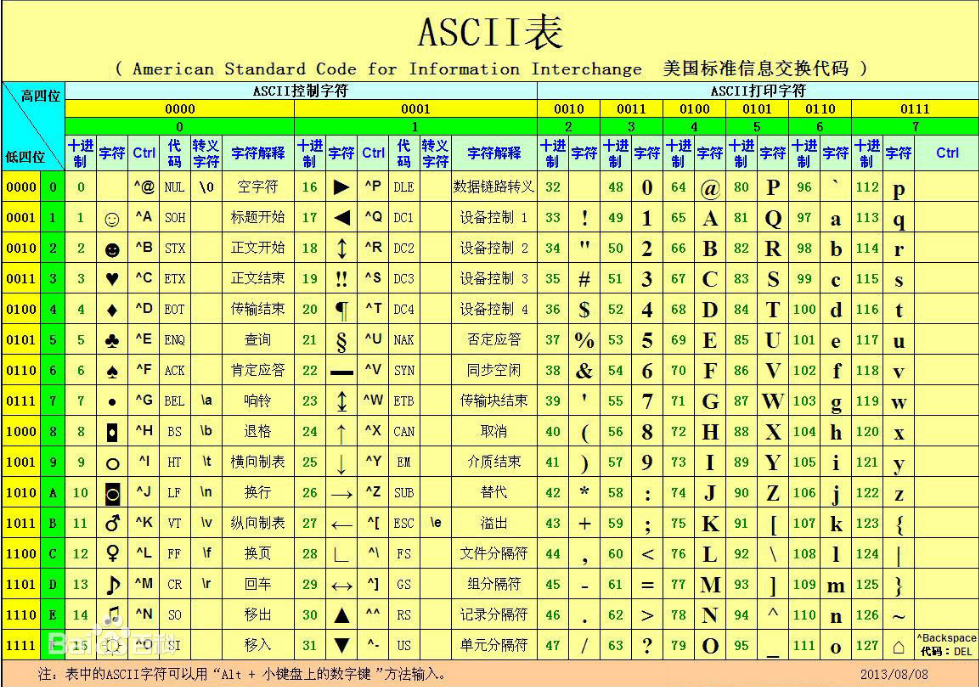ASCII表中每一个字符都需要8个bit来存储

ASCII编码的发展：

ASCII 只能存储英文和拉丁字符，一个字符占一个字节
1980年 GB2312 有 6700+ 字符
1995年 GBK1.0 有 20000+ 字符
2000年 GB18030 有 27000 中文

Unicode 万国码 支持所有国家和地区的编码
2**16 = 65535 存一个字符，同意占用 2 个字节

UTF-8 = Unicode 的扩展集，可变长的字符编码集

``````# Assic --> GB2312 --> GBK1.0 --> GB18030
# Assic --> unicode --> utf-8
``````

``````#! -*- coding:utf-8 -*-
print '我爱北京天安门'
``````

Python3.X默认支持中文。

1位 = 1bit
1字节 = 1 byte = 8 bit
abc表示三个字节，24 bit。

1 位 = 1 bit
8 bit = 1 byte = 1 字节
1024 bytes = 1 kbytes = 1 KB 1024 个字节，小文档，几百K可以表示一张图片
1024 KB = 1 Million Bbytes = 1 MB 1 兆，几万字的文档，大图片
1024 MB = 1 Gigabytes 一个清晰地电影，不是高清，高清能达到10个G
1024GB = 1　ＴＢ

1. 要具有描述性
2. 变量名只能以_，数字，字母组成。不能存在空格或特殊字符(!@#\$%&*?"~)
3. 不能以中文为变量名
4. 不能以数字开头
5. 不能使用保留字符
6. 不能以大写字母开头
7. 采用下划线命名法

input默认接收的所有数据都是字符串，即使你输入的是数字，也被当做字符串处理。

if –else缩进时，1个Tab键 != 4个空格（看起来一样，但是程序会报错）

``````score = int(input('score:'))

if score > 90:
print('A')
elif score > 80:
print('B')
elif score > 70:
print('C')
else:
print('D')
``````

• 、- 、* 、/ 、// 、% 、**
加、减、乘、除、整除、取余，次方

=、+=、-=、*=、/=、//=、**=

、>=、<=、= =、!=

and or not

“=” 赋值
“==”判断是否相等

whlie循环
while 条件：

break：终止整个循环
continue：跳出当次循环，继续下个循环

``````for i in range(1, 10):
for j in range(1, i+1):
print('%s * %s=%s' %(j, i, i*j), end='\t')
# print("{}*{}={}".format(i,j,i*j),end=" ")
print()  #换行
``````

for i in range（），就是给i 赋值
for j in range(1,i+1) ，在给i赋值的基础上，再做进一步的循环操作，即给j赋值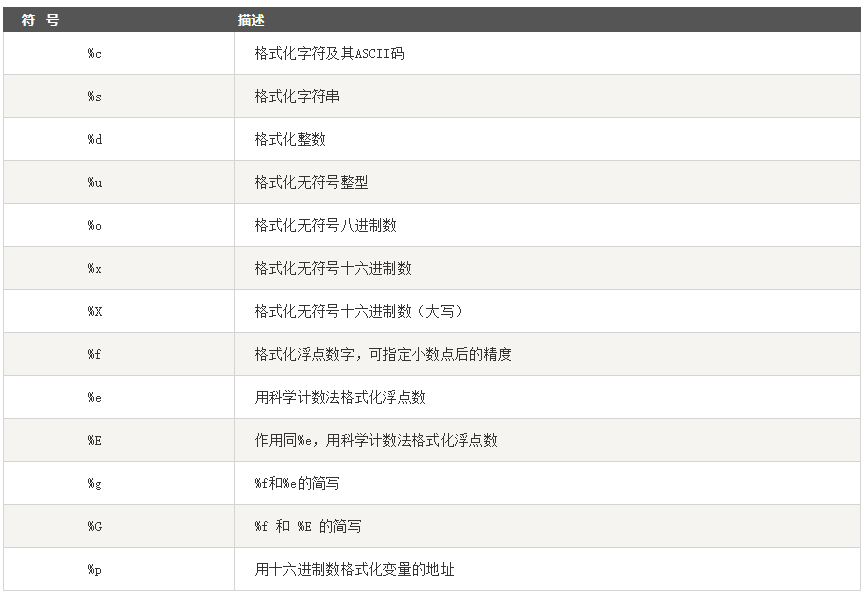format()函数：

``````print('{0} {1}'.format('hello','world'))   # hello world
print('{} {}'.format('hello','world'))    # hello world
print('{0} {1} {0}'.format('hello','world'))     # hello world hello
``````

IDE集成开发环境（IDE，Integrated Development Environment ）是用于提供程序开发环境的应用程序，一般包括代码编辑器、编译器、调试器和图形用户界面等工具。
fullstack 全栈
interpreter 解释器

``````_user = "sumatch"

for i in range(3):     #仅有三次机会
user = input("User:")
print("正确")
break      #如果输入的是对的，就跳出程序
else:
print("错误")
``````

``````exit_flag = False

for i in range(10):
if i < 5:
continue
print(i)
for j in range(10):
print('layer2:',j)
if j == 6:
exit_flag = True
break
if exit_flag:
break
``````

range(1,101,2) # 顾头不顾尾，2表示步长

list[1 : -1 : 2] # 列表中第一位到最后一位，步长为2
list[1 : -1 : -2] # 列表中最后一位到第一位，步长为2
list.append(a) 把a添加到最后面
list.insert(3,a) 把a添加到索引为3的位置
list.remove(list) 删除
del list 可以删除list,也可以删除list–>del list
list.pop(3) 删除索引为三的值，并将其返回
list.count(a) 计算a元素出现的次数
list.index(a) 返回a元素的索引值，以第一个a为主,根据内容找位置
list.reverse() 将list列表反转
list.sort() 数值从大到小排序；字符串按照ASCII码中的顺序排列
list.sort(reverse = True) 数值从小到大排序；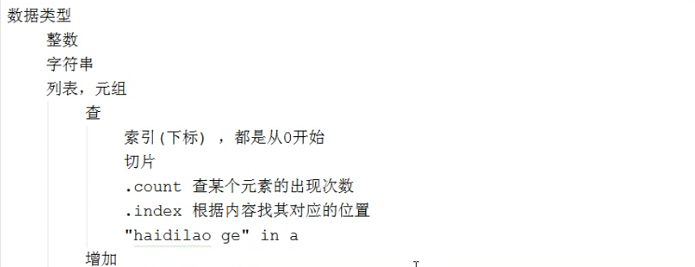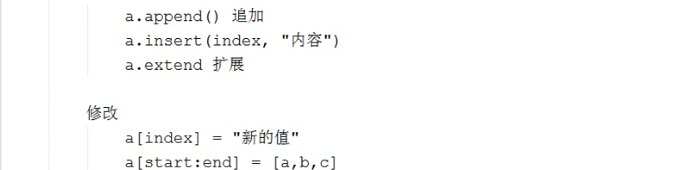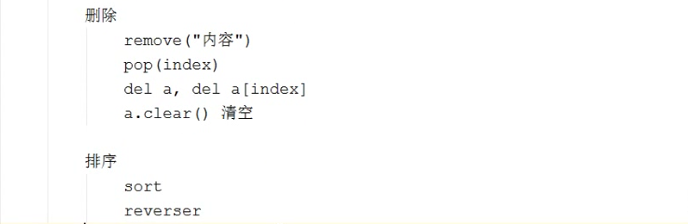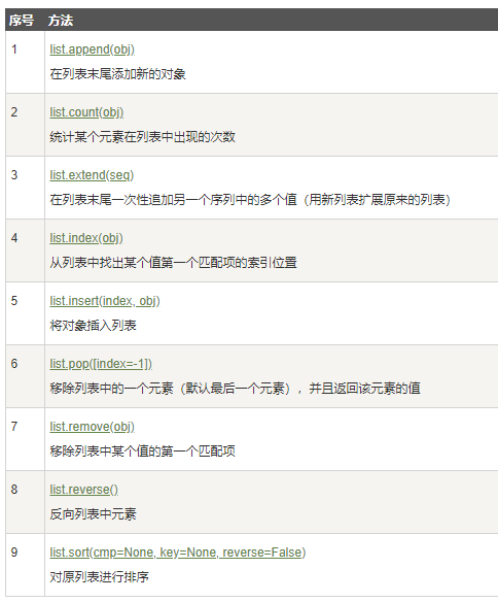a = (12,)最后一位也要加逗号

a = [2,3] 此时a是列表
a,b = [2,3] 此时a = 2，b = 3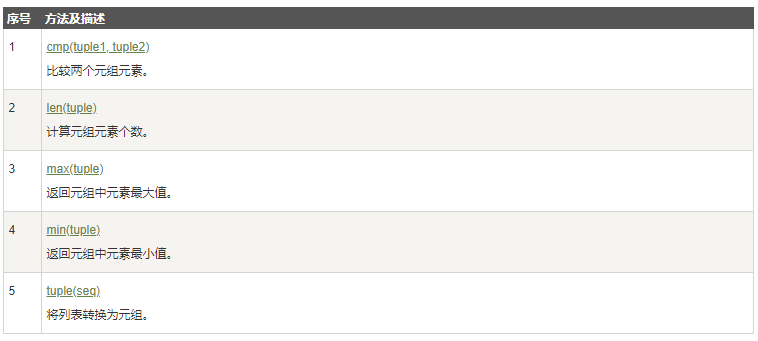dict.setdefault(key, default=None)方法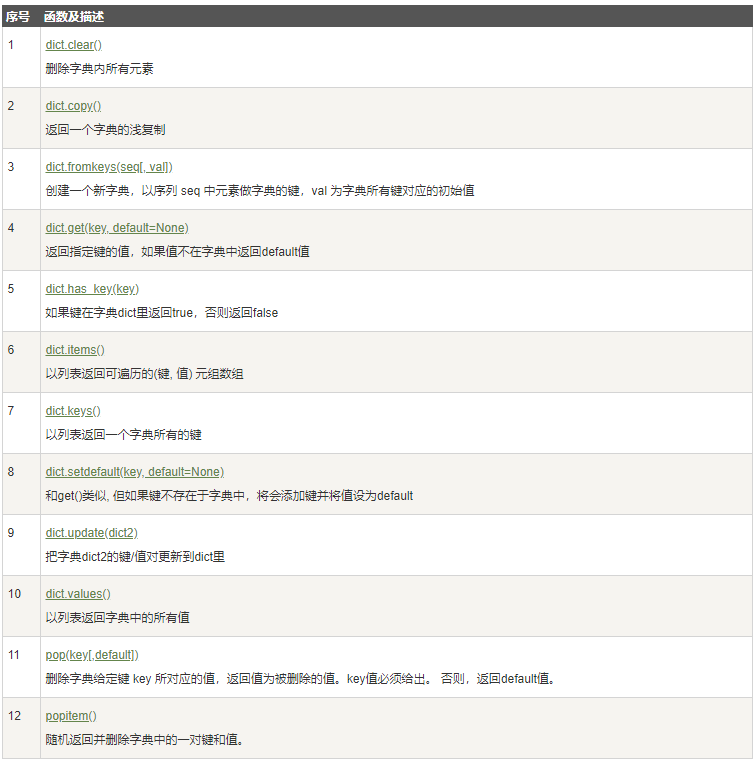str =’ ’.join(a,b) 拼接a,b，以’ ’的内容相连。
count(str, beg = 0, end = len(string)) 返回str在string中出现的次数。
replace(old, new) 替换字符串
lstrip() / rstrip() / strip() 去除字符串左/右/左右/或者指定字符
lower() 将所有大写字母转为小写
upper() 将所有小写字母转为大写
swapcase() 将所有大写字母转为小写，所有小写字母转为大写

１．可以一层一层的进入所有层
２．可以每一层返回上一层
３．可以在任一层退出　主菜单

``````menu = {
'北京':{
'朝阳':{
'国贸':{
'CICC':{},
'HP':{},
'CCTV':{},
},
'望京':{
'陌陌':{},
'奔驰':{},
'360':{},
},
'三里屯':{
'优衣库':{},
'apple':{},
},
},
'昌平':{
'沙河':{
'老男孩':{},
'阿泰包子':{},
},
'天通苑':{
'链家':{},
'我爱我家':{},
},
'回龙观':{},
},
'海淀':{
'五道口':{
'谷歌':{},
'网易':{},
'Sohu':{},
'Sogo':{},
'快手':{},
},
'中关村':{
'youku':{},
'aiqiyi':{},
'汽车之家':{},
'QQ':{},
},
},
},
'上海':{},
'山东':{},
}

parent_layers = []
while True:
for key in current_layer:
print(key)
choice = input(">>>:").strip()
if len(choice) == 0:continue
if choice in current_layer:
parent_layers.append(current_layer)
current_layer = current_layer[choice]
elif choice == "back":
if current_layer:  # []
current_layer = parent_layers.pop(-1)  # .pop()删除并返回最后一个值
else:
print("error ")
``````

.encode 编码，同时会把数据转成bytes类型
.decode解码，同时会把bytes类型转成字符串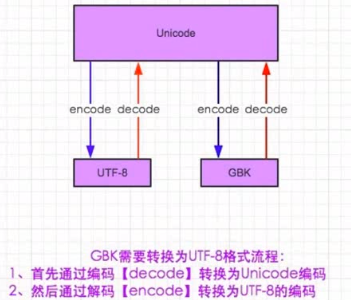open(file, mode=‘r’) 方法
Python open() 方法用于打开一个文件，并返回文件对象，在对文件进行处理过程都需要使用到这个函数，如果该文件无法被打开，会抛出 OSError。

r 以只读方式打开文件，指针放在文件开头
r+ 以读写方式打开文件，指针放在文件开头
w 打开文件用于写入，如果该文件已存在，则打开文件并从头开始编辑，原内容被删除；如果文件不存在，则创建文件开始编辑。
a 打开文件用于追加，如果该文件已存在，则打开文件并从结尾开始编辑，原内容不会被删除；如果文件不存在，则创建文件开始编辑。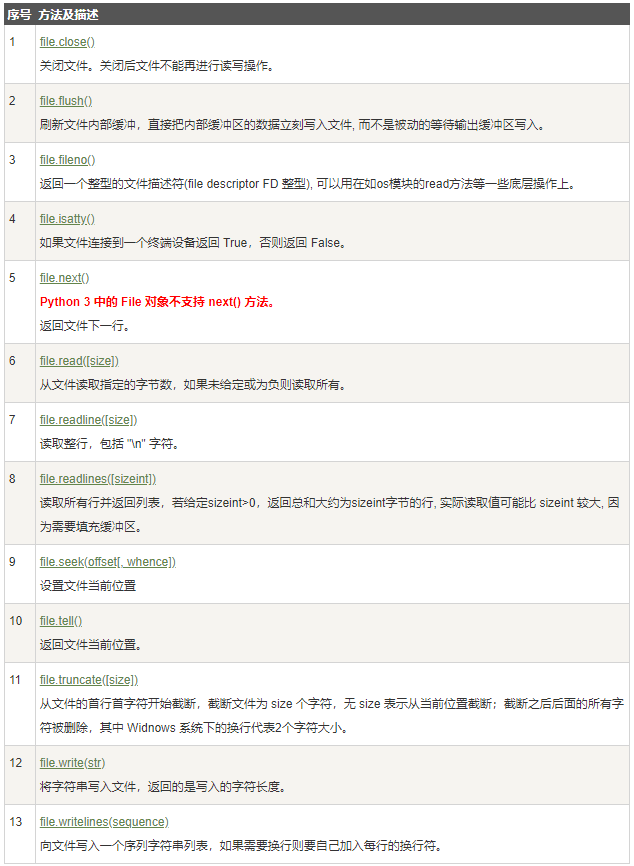``````f = open('01.txt','r')
``````

``````data = f.readlines()
f.close()

number = 0
for i in data:
number += 1
if number == 6:
i = ''.jion([i.strip(),'iiii'])  # jion 取代 +
print(i.strip())
``````

f 本身存放在磁盘中

``````for i in f:   #for循环内部将f对象做成迭代器（此时文件在磁盘中），用一个取一个（放在内存中）
print(i.strip())   #第一行打印出之后，释放内存。读取并存放第二行，打印。
``````

f.tell()方法：放回光标当前位置，可用来随时查看文件中光标的位置。

f.tell() 英文移动1个光标，中文移动3个光标
f.seek(位置)，设置光标位置，可以从任意位置进行读写操作。

f.flush 刷新文件内部缓存，将缓存中（内存中）的内容存到磁盘上去。

``````import sys,time
for i in range(30):
sys.stdout.write("*")  #往缓存中写入一个“*”，但是直到for程序执行完才打印到终端上。
time.sleep(0.2)
``````

``````import sys,time
for i in range(30):
sys.stdout.write("*")
sys.stdout.flush()  #将sys.stdout对象中缓存的“*”刷新并打印出来
time.sleep(0.2)
``````

file.truncate([size]) 截断文件

with用法：

``````f = open('log','r')
f.close()

with open('log','r') as f:
``````

``````with open('log1','r') as f1, open('log2','r') as f2:
``````

``````s1 = [[1,2],3,4]
s2 = s1
s2 = 5
s2 = "++"
print(s1)
print(s2)
``````

[[1, 5], ‘++’, 4]
[[1, 5], ‘++’, 4]

s1.copy() 将s1对象进行拷贝，返回一个s1的浅复制，只会复制第一层，两个对象之间有关联。

``````s1 = [[1,2],'22','333']
s2 = s1.copy()   #浅拷贝
s2 = 5
s2 = "++"
print(s1)
print(s2)
``````

[[1, 5], ‘22’, ‘333’]
[[1, 5], ‘++’, ‘333’]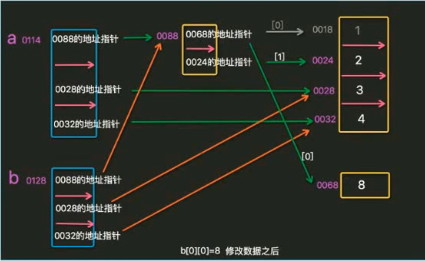``````import copy
s1 = [[1,2],3,4]
s2 = copy.deepcopy(s1)   #深拷贝
s2 = 5
s2 = "++"
print(s1)
print(s2)
``````

[[1, 2], 3, 4]
[[1, 5], ‘++’, 4]

**集合（set）**是一个无序的不重复元素序列。只能用遍历来查找里面的元素。in/not in判断元素是否在集合中。

s.update(“abc”) #将 ”a”,”b”,”c” 添加到集合中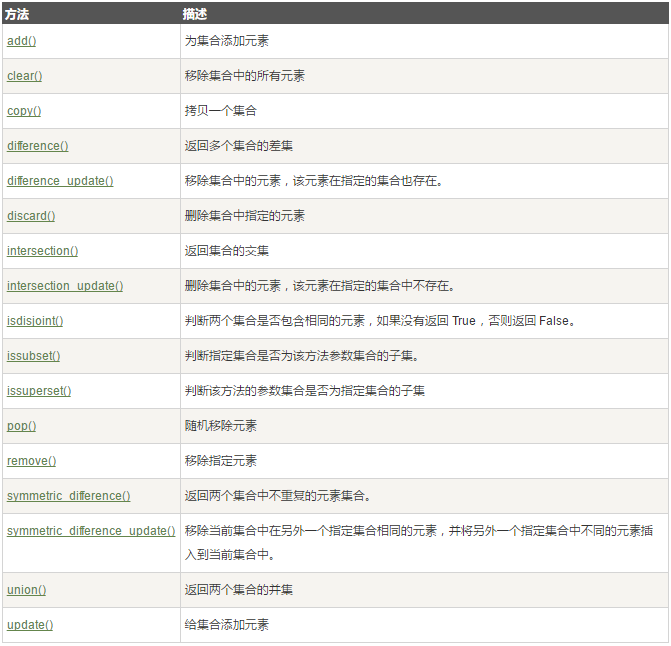set(‘alex’) == set(‘allxe’) #集合自动删除重复的元素
# set(‘alex’) 就是{‘a’, ‘l’, ‘e’, ‘x’}

• 必须参数和关键字参数:
``````def print_info(name,age):
print('Name: %s' %name)
print('Age: %d' %age)

print_info('xiaohu',39)  #必须参数
print_info(age= 39,name="xiaohu")  #关键字参数
``````
• 默认参数：默认参数要放在其他参数后面
``````def print_info(name,age,sex = 'male'):
print('Name: %s' %name)
print('Age: %d' %age)
print('sex: %s' %sex)

print_info('xiaohu',39)
print_info('lichuan',32,sex='female')
``````
• 不定长参数，*args代表不固定几个参数，将接收的参数组成一个数组。
``````def add(*args):
sum = 0
for i in args:   # args = (1, 2, 3, 4, 5)
sum += i
print(sum)
``````
• 不定长参数（二）：单值放在元组中，键值对放在字典中
``````def info(*args,**kwargs):
print(args)    #('alex', 18, 'male')
print(kwargs)   #{'hight': 175, 'job': 'it'}

info('alex',18,'male',job = 'it',hight = 175)  #无名的放前面,有名的放后面
``````

1. 结束函数，之后不得再有语句
2. 返回某个对象

1. 函数如果没有return，会返回一个None
2. 如果return多个对象，那么Python会帮我们把多个对象封装成一个元组返回

``````x = int(2.9)   # built-in
g_count = 0   # global
def outer():
o_count = 1    # enclosing
def inner():
i_count = 2   # local
print(o_count)
print(i_count)  # 错误，找不到
inner()
outer()

print(o_count) # 错误，找不到
``````

``````count = 10   #全局变量
def outer():
count = 5  #局部变量
print(count)  #往上，找局部变量。

outer()    》》》5
``````
``````count = 10   #全局变量
def outer():
print(count)  #往上，找局部变量，没有找到count,所以报错
count = 5  #局部变量

outer()   》》》报错，local variable 'count' referenced before assignment
``````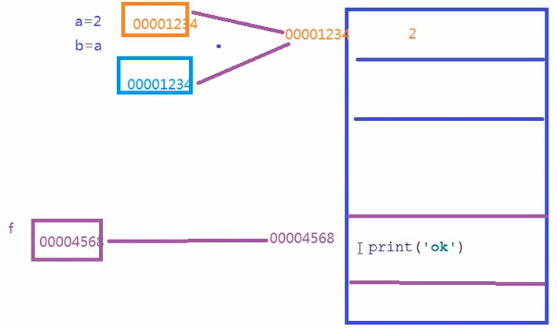``````def f(n):
return n*n

def foo(a,b,func):
return  func(a) + func(b)    # foo(1,2,f) 将f赋值给func, 即func = f ,于是返回f(a) + f(b)

foo(1,2,f)   》》》5
``````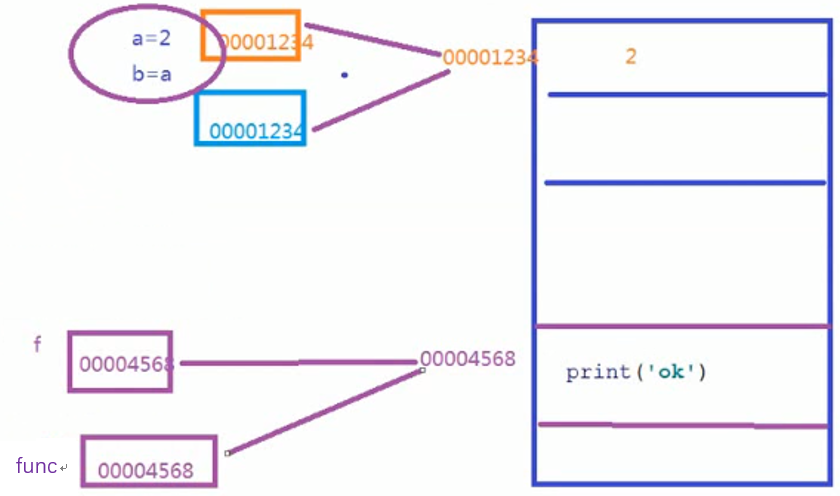``````def jc(n):
s = 1
for i in range(1,n + 1):
s *= i
return s
print(jc(1))
``````

``````def jc(n):
if n == 1:   #结束条件
return 1
return n * jc(n - 1)  #调用函数自身
print(jc(5))
``````

1. 调用函数自身
2. 必须有一个结束条件

1. filter() 函数用于过滤序列，过滤掉不符合条件的元素，返回一个迭代器对象，如果要转换为列表，可以使用 list() 来转换（py2和py3有区别）
filter(function, iterable) #function – 判断函数。iterable – 可迭代对象
``````def is_odd(n):
return n % 2 == 1

tmplist = filter(is_odd, [1, 2, 3, 4, 5, 6, 7, 8, 9, 10])
newlist = list(tmplist)
print(newlist)   》》》[1, 3, 5, 7, 9]
``````
1. map() 会根据提供的函数对指定序列做映射
map(function, iterable, …) # function 为函数， iterable 为 一个或多个序列
``````def square(x):  # 计算平方数
return x ** 2

s = map(square, [1, 2, 3, 4, 5])  # 计算列表各个元素的平方
print(list(s))
``````
1. reduce()函数
``````from functools import reduce

return x + y

``````

reduce()的作用是接收一个列表，[1,2,3,4]

1. lambda()函数，匿名函数
lambda a , b : a + b
``````from functools import reduce
print(reduce(lambda a ,b : a + b,range(1,5)))
``````

``````def outer():
x = 10
def inner():  #条件一：inner是内部函数
print(x)  #条件二：引用外部环境的一个变量
return inner  #结论：内部函数inner就是闭包
``````

• 开放：对拓展是开放的。 为什么要对扩展开放呢？
因为任何一个程序，不可能在设计之初就已经想好了所有的功能并且未来不做任何更新和修改。所以我们必须允许代码扩展、添加新功能。
• 封闭：对修改是封闭的。 为什么要对修改封闭呢？
因为我们写的一个函数，很有可能已经交付给其他人使用了，如果这个时候我们对其进行了修改，很有可能影响其他已经在使用该函数的用户。

``````import time
def foo():
start = time.time()  #记录开始时间戳
print("11233..")
time.sleep(2)
end = time.time()   #记录结束时间戳
print('spend %s'%(end - start))   #打印所用时间
foo()
``````

``````import time

def foo():
print("11233..")
time.sleep(2)

def show_time(f):
def inner():   #闭包
start = start = time.time()
f()
end = time.time()
print('spend %s' % (end - start))
return inner

foo = show_time(foo)
foo()
``````

``````def show_time(f):
def inner():   #闭包
start = time.time()
f()
end = time.time()
print('spend %s' % (end - start))
return inner

@show_time    #相当于foo = show_time(foo)
def foo():
print("11233..")
time.sleep(2)

foo()
``````

``````import time

def logger(flag = ''):  # 给装饰器加一个参数，标志位
def show_time(f):
def inner(*x,**y):   #闭包 *x,**y功能函数可传入不定长参数
start = start = time.time()
f(*x,**y)
end = time.time()
print('spend %s' % (end - start))
if flag == 'true':
print("日志记录")
return inner
return show_time

@logger('true')    #不加日志记录时，就不用传入'true’
def foo():
print("11233..")
time.sleep(2)

foo()
``````

``````a1 = [x for x in range(1,10)]
a2 = [x**2 for x in range(1,10)]
``````

``````def f(n):
return n**3

a3 = [f(x) for x in range(1,10)]
``````

``````x = [1,2,3]
for i in x:
print(i)
``````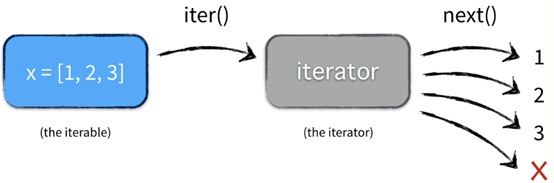1. (x*2 for x in range(5,100))
2. yield

next() 返回迭代器的下一个项目

``````a = (x*2 for x in range(5,100))   #元组
print(next(a))  #将5返回给x*2   》》》10
``````

``````def fib(max):
n, prev, curr = 0, 0, 1
while n<max:
yield curr
prev, curr = curr, curr + prev
n += 1
``````

2
f.send()，将参数传给yield前一个变量。

f.send()和next()的区别：
f.send()参数是传给yield前一个变量；next()参数是迭代器函数名字

iter() 函数返回一个迭代器。

time模块：从1970年开始

``````time.time()  #记录当前时间戳 1556723770.0009158
time.sleep(3)  #延迟3秒钟
time.localtime() #结构化时间（本地）
time.strftime('%Y-%m-%d %H:%M:%S')  #自定义时间格式2019-05-01 23:05:45
time.mktime(time.localtime())  #将本地时间转化为时间戳
``````

datetime模块

``````datetime.datetime.now()  #2019-05-01 23:18:38.512192
``````

random模块

``````import random

random.random()  # 生成一个0到1的随机数  0.758108354250769
random.randint(1, 8)  # 生成一个0到8的随机整数  4
random.choice({}/[]/()/'')  # 从一个对象中随机选择一个元素
random.randrang(1, 3)   # 从1到3中随机取一个整数（不包括3）
``````

chr() 用一个范围在 range（256）内的（就是0～255）整数作参数，返回一个对应的字符。

``````import random
def v_code():
code = ''
for i in range(5):
print(code)
v_code()
``````

os模块：与操作系统进行交互

``````import os

os.getcwd()  # 获取当前工作目录
os.chdir()   # 更改当前工作目录 change
os.makedirs('dirname1/dirname2')  # 可生成多层递归目录
os.removedirs()  #（递归）删除空文件夹
os.mkdir('dirname')  # 生成一个文件夹
os.rmdir('dirname')  # 删除一个空文件夹，若文件不为空，报错
os.remove()   #只能删除文件，不能删除文件夹
os.listdir("G:\python")  # 显示出目录下的所有文件
os.rename('oldname','newname')  # 改变文件夹名称
os.path.dirname('./abc')  # 返回abc所在的文件的路径
os.path.exists(path)  # 如果path存在，返回Ture
os.path.join(path1,path2,) # 将多个路径合并并返回
``````

sys模块：与python解释器进行交互

``````sys.argv
``````

sys.argv[]说白了就是一个从程序外部获取参数的桥梁。因为我们从外部取得的参数可以是多个，所以获得的是一个列表（list)，也就是说sys.argv其实可以看作是一个列表，所以才能用[]提取其中的元素。其第一个元素是程序本身，随后才依次是外部给予的参数。

Sys.argv[ ]其实就是一个列表，里边的项为用户输入的参数，关键就是要明白这参数是从程序外部输入的，而非代码本身的什么地方，要想看到它的效果就应该将程序保存了，从外部来运行程序并给出参数。

hashlib模块提供了很多加密的算法

``````import hashlib
def md5(arg):  # 这是加密函数，将传进来的函数加密
md5_pwd = hashlib.md5(bytes('abd', encoding='utf-8'))
md5_pwd.update(bytes(arg, encoding='utf-8'))
return md5_pwd.hexdigest()  # 返回加密后的数据（十六进制）

def log(user, pwd):  # 登陆时候的函数，由于md5不能反解，因此登陆的时候用正解
with open('db', 'r', encoding='utf-8') as f:
for line in f:
u, p = line.strip().split('|')
if u == user and p == md5(pwd):  # 登陆的时候验证用户名以及加密的密码跟之前保存的是否一样
return True

def register(user, pwd):  # 注册的时候把用户名和加密的密码写进文件，保存起来
with open('db', 'a', encoding='utf-8') as f:
temp = user + '|' + md5(pwd)
f.write(temp)

i = input('1表示登陆，2表示注册：')
if i == '2':
user = input('用户名：')
pwd = input('密码：')
register(user, pwd)
elif i == '1':
user = user = input('用户名：')
pwd = input('密码：')
r = log(user, pwd)  # 验证用户名和密码
if r == True:
print('登陆成功')
else:
print('登陆失败')
else:
print('账号不存在')
``````

logging模块：输出日志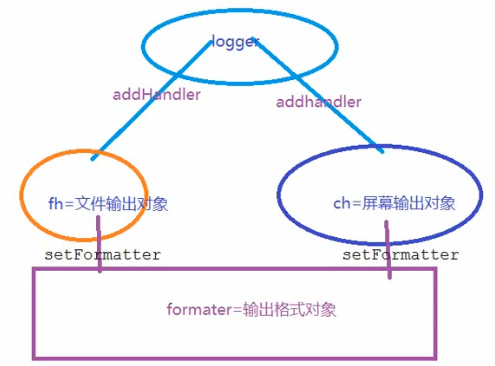``````import logging

# 1.创建一个logger
logger = logging.getLogger()  # get一个Logger对象，日志对象
logger.setLevel(logging.INFO)  # Log等级总开关

# 2.创建一个handler，用于写入日志文件
fh = logging.FileHandler('txt')  # 用于写入文件，文件对象
fh.setLevel(logging.DEBUG)  # 输出到file的log等级的开关

# 3.创建一个handler，用于输出控制台
ch = logging.StreamHandler()  # 输出控制台，屏幕对象
ch.setLevel(logging.WARNING)  # 输出到console的log等级的开关

# 4.定义handler的输出格式
formatter = logging.Formatter("%(asctime)s - %(filename)s[line:%(lineno)d] - %(levelname)s: %(message)s")

fh.setFormatter(formatter)  # fh创建格式，和formatter一样
ch.setFormatter(formatter)  # ch创建格式，和formatter一样

# 5.控制输出

# 6.日志
logger.debug('this is a logger debug message')
logger.info('this is a logger info message')
logger.warning('this is a logger warning message')
logger.error('this is a logger error message')
logger.critical('this is a logger critical message')
``````

configparser模块：用于生成和修改常见配置文件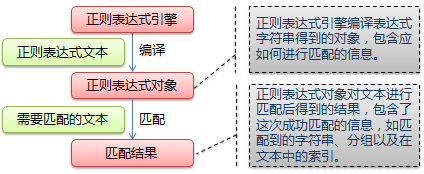``````import re

m = re.findall(r'\\','abc\de')
print(m)                # ['\\']

n = re.search(r'\bblow','blowyy')
print(n)                # <_sre.SRE_Match object; span=(0, 4), match='blow'>
``````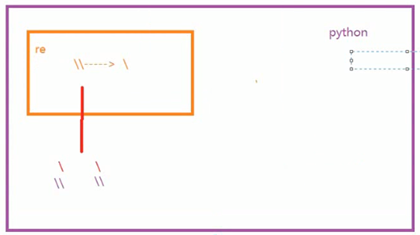re模块：正则表达式，匹配字符串
string提供的方法是完全匹配,所以需要引入正则表达式进行模糊匹配
11个元字符： . ^ \$ * + ? { } [ ] | ( ) \ 含义如下：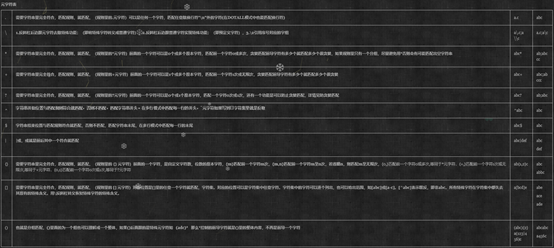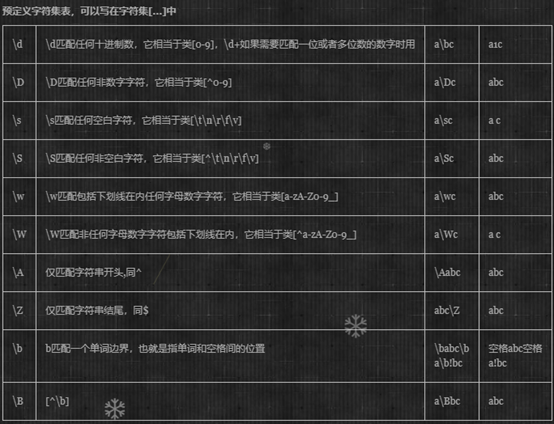1. re.findall() 遍历匹配，可以获取字符串中所有匹配的字符串，返回一个列表。
2. re.search() 匹配出第一个满足条件的字符，返回一个对象，对象可以调用group(),返回结果
3. re.match() 只在字符串开始匹配，返回一个对象
4. re.sub() 替换字符串中的匹配项
5. re.compile()用于编译正则表达式，生成一个正则表达式对象，供match()和search()函数使用

1. Python标准库
2. 第三方库
3. 应用程序自定义模块

1. import model :搜索路径sys.path,通过搜索路径找到model.py之后，python解释器将model中的所有代码（方法）解释一遍，即model = model.py里的所有代码
4. from model import add as a

``````os.path.append()   #添加路径
``````

``````os.path.dirname('./abc')  # 返回abc所在的文件的路径
os.path.abspath (__file__)  #将文件的相对路径转化为绝对路径
``````
``````if __name__ = "__main__":
``````

json
JSON (JavaScript Object Notation) 是一种轻量级的数据交换格式。
Python3 中可以使用 json 模块来对 JSON 数据进行编解码，它包含了两个函数：
json.dumps(): 对数据进行编码。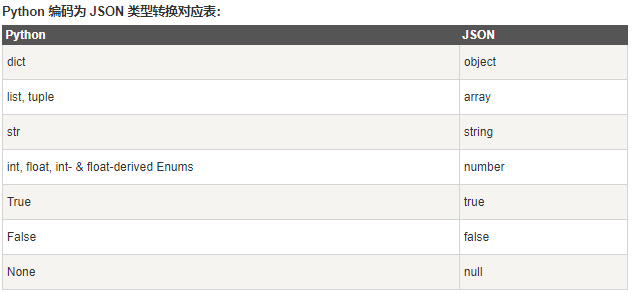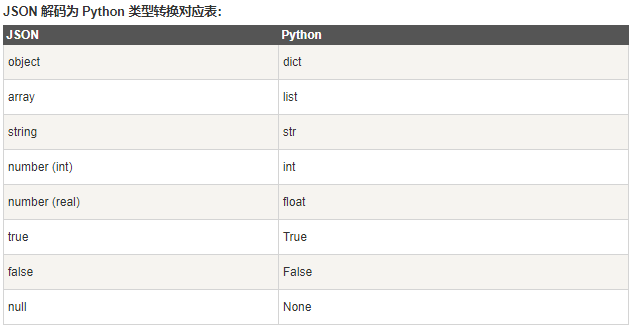python的两种编程思想：1.面向对象编程 2.函数式编程

``````def foo(name, age, gender, content):
print(name, age, gender, content)

foo(小明，10岁，男，上山去砍柴)
foo(小明，10岁，男，开车去东北)
foo(小明，10岁，男，最爱打篮球)
``````

``````class Bar：
def foo(name, age, gender, content):
print(name, age, gender, content)

obj = Bar()
obj. foo(小明，10岁，男，上山去砍柴)
obj.foo(小明，10岁，男，开车去东北)
obj.foo(小明，10岁，男，最爱打篮球)
``````

``````def + 函数名（参数）
``````

``````class + 类名：
def ：方法，方法中第一个参数必须是self
``````

obj = Bar() #创建中间人（称为对象，实例）
obj.foo()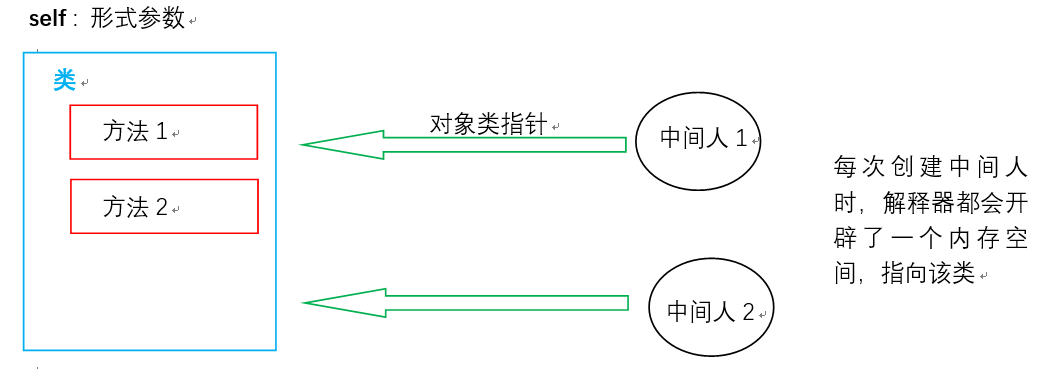self代指调用方法的中间人（实例，对象）

``````class Bar:

def foo(self,arg):
print(self,arg)

z1 = Bar()
print(z1)      # <__main__.Bar object at 0x0000029AC8AAA470>
z1.foo(111)     # <__main__.Bar object at 0x0000029AC8AAA470> 111

z2 = Bar()
print(z2)    # <__main__.Bar object at 0x0000029AC8AAA4E0>
z2.foo(666)    # <__main__.Bar object at 0x0000022CF6C7A4E0> 666
``````

``````class Bar:
def __init__(self):    # 称之为构造方法
print("1122")

def foo(self):   # 普通方法
print('5555')

z1 = Bar()   # 创建中间人时，编译器会自动执行__init__方法
z1.foo()    # 调用foo()方法
``````

1.如何创建类

class 类名：
pass

2.创建方法

obj = 类(‘al’)

obj = 类(‘xx’)
obj.普通方法名()

3.面向对象三大特性之一：封装

``````class Bar:
def __init__(self,n,a):   #所有方法的共同属性
self.name = n
self.age = a
self.xuexing = 'o'   #默认所有人的血型为 o
b1 = Bar('alex',13)
b2 = Bar('weer',15)
``````

4.适用场景

``````class F:
def f1(self):
print("111")
def f2(self):
print('556')

class S(F):
def s1(self):
print('888')
def f2(self):    #重写可防止执行父类中的该方法
print('666')
#如果此时想执行父类中的f2方法，有两种方式
super(S,self).f2()  #方式一
F.f2(self)     #方式二

obj = S()   #如果有init方法，只执行一次
obj.s1()  #888
obj.f2()  #666 /n 556
``````

python中支持多继承

1. 左侧优先
2. 一条道走到黑
3. 有同一根时，根最后执行

python原生多态。

1. 字段
• 普通字段，保存在对象中，执行只能通过对象访问
• 静态字段，保存在类中，执行时既可以通过对象访问，也可以通过类访问
1. 方法（保存在类中）
• 普通方法，执行时通过对象调用 self>>对象
• 静态方法，需要加装饰器（@staticmethod），执行通过类直接调用
• 类方法，需要加装饰器（@classmethod），执行通过类直接调用 cls>>当前类
``````class Province:
# 静态字段，属于类
country = '中国'

def __init__(self,name):
# 普通字段，属于对象
self.name = name
``````

``````class Foo:
# 普通方法
def bar(self):
print('bar')

# 静态方法，self非必须，可不传参数
@staticmethod
def sta():
print('123')

#静态方法，可以传参数
@staticmethod
def stat(a1,a2):
print(a1,a2)

Foo.sta()   >>>123
Foo.stat(1,5)   >>>1,5
``````

``````#属性：普通方法加装饰器（@property）
``````

``````class Foo:

@property  #属性
def bar(self):
return 1

obj = Foo()
r = obj.bar  #通过创建对象调用，但是不需要加括号
print(r)   >>>1
``````

``````class Foo:

@property  #属性,用于执行obj.bar
def bar(self):
return 1

@bar.setter  # 用于执行obj.bar = 123
def bar(self,val):
print(val)

obj = Foo()
r = obj.bar  #通过对象调用，但是不需要加括号
print(r)
obj.bar =123
``````

``````class Pergination:
def __init__(self,current_page):
try:
p = int(current_page)
except Exception as e:
p = 1
self.page = p

@property  # 为了后续调用不需要再加括号
def start(self):
val = (self.page -1)*10
return val

@property
def end(self):
val = self.page * 10
return val

li = []
for i in range(1000):
li.append(i)

while True:
page = input('请输入要查看的页码：')   # 每页显示10条
obj = Pergination(page)
# print(li[obj.start():obj.end()])  # 如果不加装饰器，此处需加括号
print(li[obj.start:obj.end])
``````

1.字段私有

``````class Foo:
def __init__(self,name,age):
self.name = name
self.__age = age  # 私有，外部无法直接访问

obj = Foo('aaa',19)
print(obj.name)    >>>aaa
print(obj.__age)   >>>'Foo' object has no attribute '__age'
``````

``````class Foo:
__v = '112'  # 私有，外部无法直接访问
def __init__(self,name,age):
self.name = name
self.__age = age  # 私有，外部无法直接访问

@staticmethod
def show():
return Foo.__v

print(Foo.show())   >>>112
obj = Foo('aaa',19)
print(obj.name)   >>>aaa
``````

2.方法私有

``````class Foo:

def __f1(self):  # 方法名前加下划线，私有
return 123

obj = Foo()
print(obj.f2())   >>>'Foo' object has no attribute 'f2'
``````

3.静态方法和类方法也是同样方法私有

``````__init__   类(), 自动执行
__call__   对象() 或者 类()(), 自动执行
__int__    int(对象), 获取返回值，并赋值给对象
__str__    str(对象)，获取返回值，并赋值给对象
__dict__   将封装中封装的所有内容通过字典返回
``````
``````class Foo:

def __init__(self):   # 类+()  执行
print('init')

def __call__(self, *args, **kwargs):  # 对象+()  执行
print('call')

obj = Foo()  >>>init
obj()   >>>call
``````
``````__getitem__  ，切片或者索引，li或者li[1:5:2]调用，将8当作参数传入
__setitem__   li = 'fgds' ，将索引为100的值设置为'fgds'
__delitem__  del li，删除对应索引的值
``````
``````class Foo:

def __init__(self):   # 类+()  执行
print('init')

def __getitem__(self, item):   # 查询
return item + 10

def __setitem__(self, key, value):  # 设置，无需返回值
print(key,value)

def __delitem__(self, key):   # 删除，无需返回值
print(key)

li = Foo()  # >>>init
r = li   # 自动执行li对象的类中的 __getitem__方法，8 当作参数传递给items
print(r)   # >>>18
li = 'fgds'   # 设置对应索引的值
del li  #删除对应索引的值
``````
``````__iter__ :   for循环调用执行
如果类中有__iter__方法，对象将变成可迭代器
对象.__iter__ 的返回值：迭代器
for循环：
1.执行对象li的类Foo中的__iter__方法，并获取器返回值
2.循环上一步返回的对象
``````
``````class Foo:

def __init__(self):   # 类+()  执行
print('init')

def __iter__(self):
return iter([11,22,33])

li = Foo()  # >>>init
for i in li:
print(i)   #>>>11 换行 22  换行 33
``````

python中，一切皆对象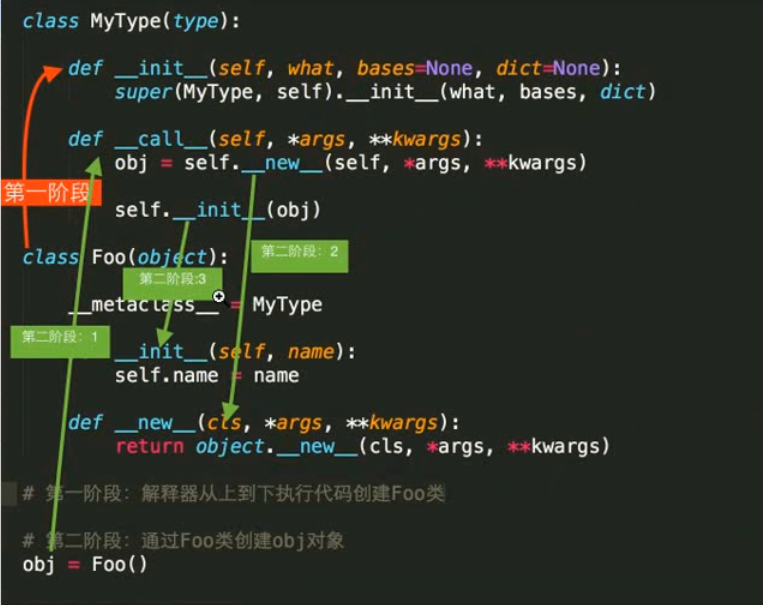``````try:
# 代码块，逻辑
except Exception as e:
# 上述代码块如果出错，执行这里内容
# 可以print(e)查看错误信息
``````

e是Exception的对象，对象中封装了错误信息

assert 条件

getattr()函数用于返回一个对象属性值。'name’是字符串。也可反射类和模块。
hasattr()判断对象中是否含有某属性
setattr(obj，‘sex’, ‘men’) 给obj对象添加属性sex，值为men
delattr(obj,‘name’) 删除obj对象中的name属性

``````class Foo:
def __init__(self,name,age):
self.name = name
self.age = age

obj = Foo('Alex',18)
print(getattr(obj,'name'))  # >>> Alex
print(hasattr(obj,'name'))  # >>> True
setattr(obj,'sex','men')
print(obj.sex)     # >>> men
delattr(obj,'name')
``````

web框架中，有很多URL用到getattr()函数，因为这个函数功能很强大，可以反射类和模块。

obj = Foo() # obj对象，也称为Foo类的实例（实例化）。

obj 1 = Foo()
obj 2 = Foo()
obj 3 = Foo()

python实现单例模式的代码：通过类方法和静态字段伪造出一个每次创建时都只构造一个实例的模式，由下面代码可以看出再次创建obj2时候，使用的还是obj1的内存地址。

``````class Foo:
__v = None

@classmethod    # 这个装饰器的第一个参数必须是cls
def get_instance(cls):
if cls.__v:
return cls.__v
else:
cls.__v = Foo()
return cls.__v

obj1 = Foo.get_instance()   # @classmethod通过类直接调用
print(obj1)     # >>> <__main__.Foo object at 0x000001D4BFCA29B0>
obj2 = Foo.get_instance()
print(obj2)     # >>> <__main__.Foo object at 0x000001D4BFCA29B0>
``````

1 0

pdf(new) 更多>